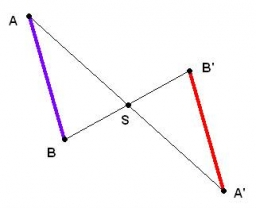# Trapezium diagonals

It is given trapezium ABCD with bases | AB | = 12 cm, |CD| = 8 cm. Point S is the intersection of the diagonals for which |AS| is 6 cm long. Calculate the length of the full diagonal AC.

x =  10 cm

### Step-by-step explanation:Did you find an error or inaccuracy? Feel free to write us. Thank you!Tips to related online calculators
Check out our ratio calculator.

#### You need to know the following knowledge to solve this word math problem:

We encourage you to watch this tutorial video on this math problem:

## Related math problems and questions:

• Trapezoid - intersection of diagonalsIn the ABCD trapezoid is AB = 8 cm long, trapezium height 6 cm, and distance of diagonals intersection from AB is 4 cm. Calculate the trapezoid area.
• Rectangular trapezoidThe ABCD rectangular trapezoid with the AB and CD bases is divided by the diagonal AC into two equilateral rectangular triangles. The length of the diagonal AC is 62cm. Calculate trapezium area in cm square and calculate how many differs perimeters of the
• Diagonal intersectisosceles trapezoid ABCD with length bases | AB | = 6 cm, CD | = 4 cm is divided into 4 triangles by the diagonals intersecting at point S. How much of the area of the trapezoid are ABS and CDS triangles?
• Trapezoid IVIn a trapezoid ABCD (AB||CD) is |AB| = 15cm |CD| = 7 cm, |AC| = 12 cm, AC is perpendicular to BC. What area has a trapezoid ABCD?
• Diagonals at right angleIn the trapezoid ABCD, this is given: AB=12cm CD=4cm And diagonals crossed under a right angle. What is the area of this trapezoid ABCD?
• DiagonalThe rectangular ABCD trapeze, whose AD arm is perpendicular to the AB and CD bases, has an area of 15 cm square. Bases have lengths AB = 6cm, CD = 4cm. Calculate the length of the AC diagonal.
• Trapezoidal prismCalculate the surface of the quadrilateral prism ABCDA'B'C'D 'with the trapezoidal base ABCD. The height of the prism is 12 cm; ABCD trapezoidal data: AB base length is 8 cm, CD base length is 3 cm, BC arm length is 4 cm, and AC diagonal length is 7 cm. L
• IS trapezoidCalculate the length of diagonal u and height v of isosceles trapezoid ABCD, whose bases have lengths a = |AB| = 37 cm, c = |CD| = 29 cm and legs b = d = |BC| = |AD| = 28 cm.
• Isosceles trapezoidIn an isosceles trapezoid KLMN intersection of the diagonals is marked by the letter S. Calculate the area of trapezoid if /KS/: /SM/ = 2:1 and a triangle KSN is 14 cm2.
• Rectangular trapezoidThe rectangular trapezoid ABCD is: /AB/ = /BC/ = /AC/. The length of the median is 6 cm. Calculate the circumference and area of a trapezoid.
• Trapezoid RTThe plot has a shape of a rectangular trapezium ABCD, where ABIICD with a right angle at the vertex B. side AB has a length of 36 m. The lengths of the sides AB and BC are in the ratio 12:7. Lengths of the sides AB and CD are a ratio of 3:2. Calculate con
• Draw a trapezoidDraw a trapezoid if given a = 7 cm, b = 4 cm, c = 3.5 cm, diagonal AC = 5cm. Solve as a construction task.
• Isosceles trapezoidIsosceles trapezoid ABCD, AB||CD is given by |CD| = c = 12 cm, height v = 16 cm and |CAB| = 20°. Calculate area of the trapezoid.
• Trapezium ABCDIn the figure, ABDC is a trapezium in which AB || CD. line segments RN and LM are drawn parallel to AB such that AJ=JK=KP. If AB=0.5m and AP=BQ=1.8m, find the lengths of AC, BD, RN and LM. angle D=angle C=60
• Similarity coefficientIn the triangle TMA the length of the sides is t = 5cm, m = 3.5cm, a = 6.2cm. Another similar triangle has side lengths of 6.65 cm, 11.78 cm, 9.5 cm. Determine the similarity coefficient of these triangles and assign similar sides to each other.
• Trapezoid - diagonalA trapezoid has a length of diagonal AC crossed with diagonal BD in the ratio 2:1. The triangle created by points A, cross point of diagonals S and point D has area 164 cm2. What is the area of the trapezoid?
• Rhombus constructionConstruct ABCD rhombus if its diagonal AC=9 cm and side AB = 6 cm. Inscribe a circle in it touching all sides...